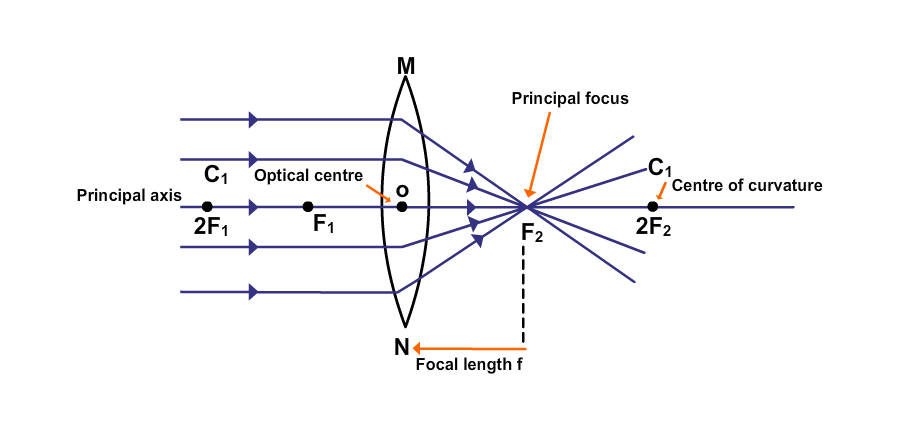Convex Lens Focal Length

# Our Objective

To determine the focal length of a thin convex lens by obtaining the image of a distant object.

# The Theory

## Convex Lens

A convex lens is a lens formed by two spherical surfaces bulging outward. It converges a beam of light incident on it and hence is known as a converging lens. Convex lenses are thicker in the middle and thinner at the edges. The light rays when refracted through a convex lens obey the laws of refraction.

## Basic lens parameters

• O = optical centre of the lens.
• F1, F2= Foci of the lens.A lens has a focus on either side.
• 2F1, 2F2= Points that lie at a distance twice that of the focal length from O
• f =focal length of the lens (distance between O and F1 or O and F2)
• C1, C2 = Centre of curvature of the lens## Centre of curvature

The two spherical surfaces of a lens forms a part of a sphere. The centers of these spheres are called centres of curvature denoted as C1 and C2.

## Principal Axis

The principal axis is an imaginary straight line passing through the two centres of curvature corresponding to the spherical surfaces of the convex lens.

## Optical Centre

A point in the centre of the lens lying on the principal axis is said to be the optical centre of the lens. A ray of light passing through the optical centre does not suffer any deviation.

## Focus

A beam of light parallel to the principal axis of the convex lens passes through the lens and converges at a point called the principal focus. Convex lenses have two foci, one for each spherical surface. The focus on the side of the light source is called the first focal point, whereas the other is called the second focal point. For an equiconvex lens, the two foci are equidistant from the optical centre. The distance between the optical centre and one of the focus points is called the focal length of the lens.

The rays of light coming from a distant object such as the sun (or a distant tree or a distant building) can be considered to be parallel to each other. These rays enter the lens and after refraction converge at the focus on the other side of the lens. The real and inverted image of the distant object formed at the focus of the lens can be captured on a screen. Thus, the distance between the lens and the screen corresponds to the focal length of the convex lens.

## Power of a lens

It is the ability of a lens to converge or diverge the rays passing through it. Unit of power is dioptre (D). It is related to the focal length f expressed in metres as -

P =1 ∕ f

# Learning Outcomes

• Students learn the basic features of a convex lens.
• Image formation by a convex lens of a distant object is analyzed.
• Students learn to find the approximate focal length of an unknown convex lens.